Question

Three exterior angles of a pentagon measures 60,80and 90.Find the measure of other two exterior angle, assuming them equally Thanks!​

1.bonexptip

Given:

The three exterior angles of a pentagon measures 60,80 and 90.

To find:

The measure of other two exterior angle, assuming them equally.

Solution:

Let x be the measure of two other exterior angles of the pentagon.

We know that the sum of all exterior angles of a pentagon is 360 degrees.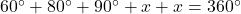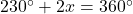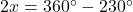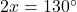Divide both sides by 2.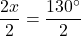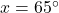Therefore, the measures of both exterior angles are 65 degrees.